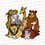# Elementary Techniques used in the IMO (International Mathematical Olympiad) - Invariants

This is continuation from Post 8. For readers who have read this, please do NOT post your solutions in the other post, instead post it in the comments below in THIS post. Thank you.

$\LARGE \text{Hints}$

1. Well, since we cannot spam this directly, consider working backwards. Clearly, Tom needs to ensure that the buckets have $a_1,\ldots,a_5\le 1$ or else the bucket would overflow on Jerry's turn. Suppose then that Jerry adds $w_1,\ldots,w_5$ to the buckets, where $w_1+\cdots+w_5=1$. So if we think about it further, we can see that if there are $2$ nonadjacent buckets (we are trying to make Jerry win now), Jerry wins if we have $a_i + w_i > 1$ and $a_{i+2} + w_{i+2} > 1$. Tom needs to prevent Jerry from setting $(w_i,w_{i+2})=(1+\epsilon-a_i,1+\epsilon-a_{i+2})$, so all he does is to ensure that $2+2\epsilon-a_i-a_{i+2}>1=w_1+\cdots+w_5$ for all $\epsilon>0 \rightarrow a_i+a_{i+2}\le 1$. Now it is just your job to prove that these invariants hold at every step.

2. There isn't a really detailed hint for this question. Remark first that: If we start with a point on the convex hull of $\mathcal{S}$ and a line $\ell$ that is “tangent” to the convex hull then we will only iterate over the points from the convex hull. The key motivation behind the solution is the fact that in rotations there are some really important angles such as $\pi, \frac{\pi}{2}$. Also think, if we draw a line $\ell$, then the plane is split into two sections and there is one point only on $\ell$, how do we know that we can iterate? Maybe we can see what happens if we swap the two sections? Do you see the invariance?

3. Clearly we need to construct a monovariant. Think: Is $2013^{2013}$ purposely there to put you off? Try some small cases. It becomes apparent that we need an alternating odd even structure. Prove that this is possible. Try casework if everything else fails. We need a monovariance that looks something like: $max\{ a,b,c \} - g(a,b,c)$ Here we define $g(a,b,c) = \text{\# of} \space a,b,c \space \text{that are equal to} \space \max \{ a,b,c \}$. The alternating structure suggests strongly to use $3 max\{ a,b,c \} - g(a,b,c)$. Use induction to prove that this invariance holds a every step.Note by Anqi Li
7 years, 5 months ago

This discussion board is a place to discuss our Daily Challenges and the math and science related to those challenges. Explanations are more than just a solution — they should explain the steps and thinking strategies that you used to obtain the solution. Comments should further the discussion of math and science.

When posting on Brilliant:

• Use the emojis to react to an explanation, whether you're congratulating a job well done , or just really confused .
• Ask specific questions about the challenge or the steps in somebody's explanation. Well-posed questions can add a lot to the discussion, but posting "I don't understand!" doesn't help anyone.
• Try to contribute something new to the discussion, whether it is an extension, generalization or other idea related to the challenge.
• Stay on topic — we're all here to learn more about math and science, not to hear about your favorite get-rich-quick scheme or current world events.

MarkdownAppears as
*italics* or _italics_ italics
**bold** or __bold__ bold
- bulleted- list
• bulleted
• list
1. numbered2. list
1. numbered
2. list
Note: you must add a full line of space before and after lists for them to show up correctly
paragraph 1paragraph 2

paragraph 1

paragraph 2

[example link](https://brilliant.org)example link
> This is a quote
This is a quote
    # I indented these lines
# 4 spaces, and now they show
# up as a code block.

print "hello world"
# I indented these lines
# 4 spaces, and now they show
# up as a code block.

print "hello world"
MathAppears as
Remember to wrap math in $$ ... $$ or $ ... $ to ensure proper formatting.
2 \times 3 $2 \times 3$
2^{34} $2^{34}$
a_{i-1} $a_{i-1}$
\frac{2}{3} $\frac{2}{3}$
\sqrt{2} $\sqrt{2}$
\sum_{i=1}^3 $\sum_{i=1}^3$
\sin \theta $\sin \theta$
\boxed{123} $\boxed{123}$

## Comments

There are no comments in this discussion.

×

Problem Loading...

Note Loading...

Set Loading...